## HMTA Piano & String Competition

 /* styles */ The annual Hawaii Music Teachers Association competition was held on October 22, 2017 at the U.H. Manoa Music Department. It was an all-day affair involving performances on violin, viola, cello and piano by students ages 5-18. Several teachers from Masaki School of Music (MSM) volunteered their time to organize and help out during the competition. As always, the student performances were excellent. Students from MSM had some stiff competition but came out ahead in more ways than one. In our eyes, they are all winners! Every single participant worked diligently with their teacher and, as a result, have elevated their playing to a new level. Bravo to all!
 table div table+table+table+table+table div table td,table.module-4{width:100%;padding:0}table div table+table+table+table+table div table{width:100%;float:none;margin-left:auto;margin-right:auto;padding:0}table div table+table+table+table+table div table a{border:0 none;text-decoration:none}table div table+table+table+table+table div table img{width:100%!important;border:0 none;text-decoration:none}/* styles */
 /* styles */ There were 11 students representing MSM. Students of Alice Yeung, Seon Choi, Rosy Wang, Dr. Tommy Yee and Nancy Masaki were among those competing. We are pleased to announce the winners: Brix Kozuki, student of Seon Choi, placed 3rd in Level 3 on piano Madeline Hodge, student of Rosy Wang, placed 1st in Level 3 on piano Shawn Jun, student of Dr. Tommy Yee, placed 3rd in Level 4 on piano Pyotr Alvarado, student of Nancy Masaki, placed 2nd in Level 2 on cello Celina Lim, student of Nancy Masaki, placed 1st in Level 3 on cello Mira Hu, student of Nancy Masaki, placed 2nd in Level 4 on cello
 table div table+table+table+table+table+table+table div table{width:100%;padding:0}table div table+table+table+table+table+table+table div table img{width:96.23%;padding:0;float:none}table div table+table+table+table+table+table+table div table td{width:100%;padding:0 1.88% 18px}/* styles */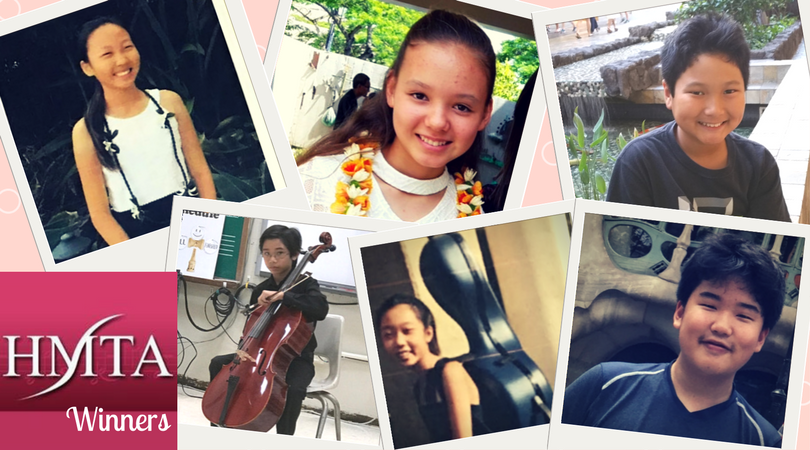From top left: Celina Lim, Madeline Hodge, Brix Kozuki, Pyotr Alvarado, Mira Hu, Shawn Jun
 table div table+table+table+table+table+table+table+table+table div table{width:100%;padding:0}table div table+table+table+table+table+table+table+table+table div table img{width:96.23%;padding:0;float:none}table div table+table+table+table+table+table+table+table+table div table td{width:100%;padding:0 1.88% 18px}/* styles */## MTNA Competitions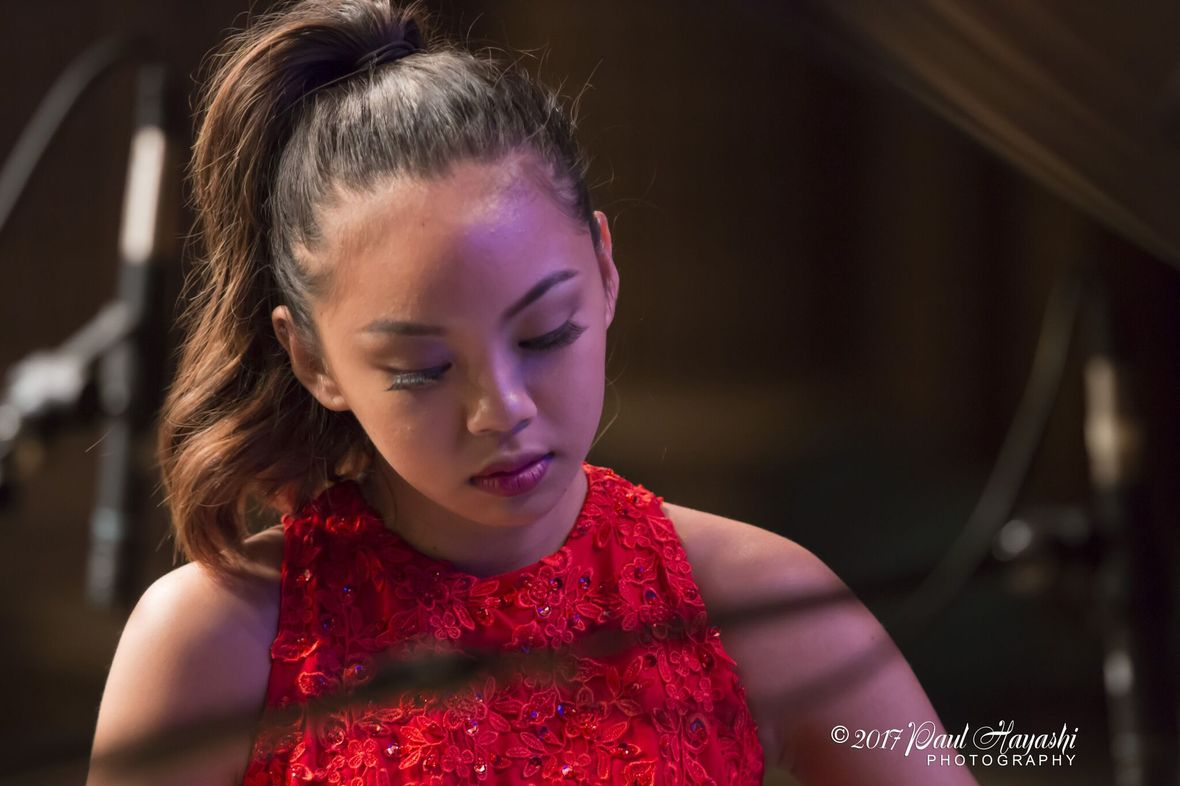Photo credit: Paul Hayashi Photography

The Music Teachers National Association (MTNA) will hold a competition on Sunday, October 29th. Representing Hawaii in the Regional Competition Young Artist Piano Performance that will be held in Las Vegas January 5-7, 2018 will be Noe Baladad, student of Dr. Tommy Yee. Results for the Junior String, Senior String, Junior Piano and Senior Piano have yet to be determined.

 /* styles */ If you are interested in attending the remaining MTNA Competitions this Sunday, October 29th, admission is free and open to the public at the U.H. Music Department. The MTNA Piano Competition for Junior, Senior, Duet and Young Artist will be held in Orvis Auditorium: 8:00am - Senior Piano Duet performance 8:30am - Young Artist Piano Representative, Noe Baladad Please come to support Noe! 9:05am - Junior Piano Competition 11:10am - Senior Piano Competition The MTNA Junior and Senior String competitions will be at the following times: 12:30pm - Junior Strings, room 212 2:00pm - Senior Strings, room 212
 table div table+table+table+table+table+table+table+table+table+table+table+table+table div table{width:100%;padding:0}table div table+table+table+table+table+table+table+table+table+table+table+table+table div table img{width:96.23%;padding:0;float:none}table div table+table+table+table+table+table+table+table+table+table+table+table+table div table td{width:100%;padding:0 1.88% 18px}/* styles */## HMTA / MTNA Celebration Recital this Sunday at 7:00pm

 /* styles */ To celebrate the hard work and courageous effort all the participants put into preparing for this competition, we will be featuring all of the Masaki School of Music participants in a recital at the Masaki School of Music this Sunday, October 29th at 7:00pm. Admission is free.
 table div table+table+table+table+table+table+table+table+table+table+table+table+table+table+table+table div table{width:100%;padding:0}table div table+table+table+table+table+table+table+table+table+table+table+table+table+table+table+table div table img{width:96.23%;padding:0;float:none}table div table+table+table+table+table+table+table+table+table+table+table+table+table+table+table+table div table td{width:100%;padding:0 1.88% 18px}/* styles */## Madeline Schatz-Harris Youth Concerto Competition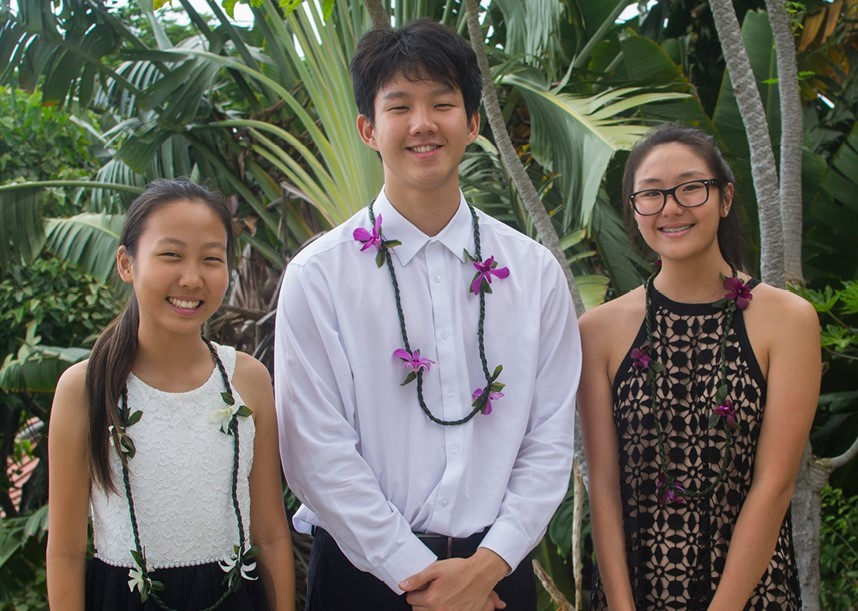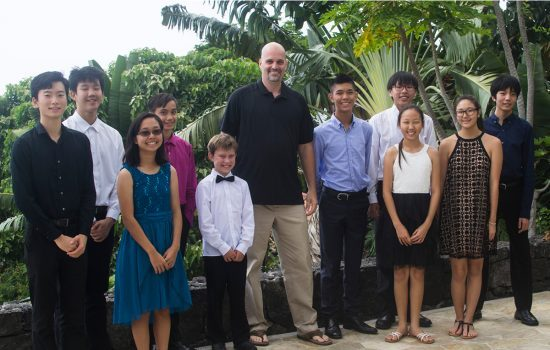/* styles */ The Kamuela Philharmonic recently announced, "We are very pleased to introduce the winners of our annual Madeline Schatz-Harris Youth Concerto Competition, which took place on Saturday, September 9, 2017, at a private estate in Kailua-Kona. Young string instrument players from around the Aloha State were eligible to compete this year. During the competition, student musicians from three age categories (12 years and under, 13–15 years, and 16–18 years) performed, by memory, one movement of a piece chosen from standard orchestral repertoire with piano accompaniment." "All ten contestants performed so well it was difficult for judges to select the winners, who are cellist Celina Lim, 11, violinist Allyson Kwong, 14, and cellist Joshua Chang, 18. These three winners will perform their solo works in concert at Kahilu Theatre in Waimea, accompanied by the Kamuela Philharmonic Orchestra, on Sunday, January 28, 2018." "Young Musicians Dazzle During our Annual Youth Concerto Competition". Kamuela Philharmonic, Blog. http://www.kamuelaphil.org/young-musicians-dazzle/
 table div table+table+table+table+table+table+table+table+table+table+table+table+table+table+table+table+table+table+table+table div table{width:100%;padding:0}table div table+table+table+table+table+table+table+table+table+table+table+table+table+table+table+table+table+table+table+table div table img{width:96.23%;padding:0;float:none}table div table+table+table+table+table+table+table+table+table+table+table+table+table+table+table+table+table+table+table+table div table td{width:100%;padding:0 1.88% 18px}/* styles */## Early Childhood Music Program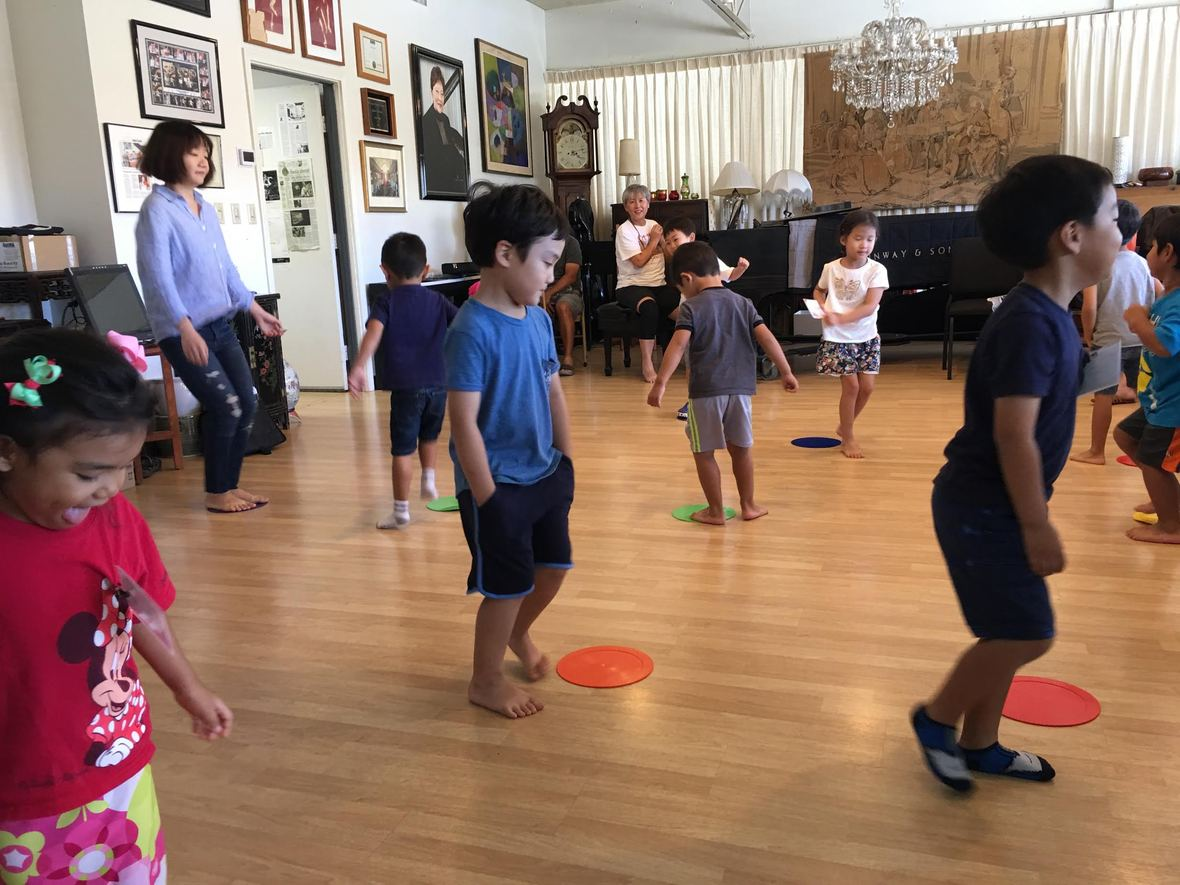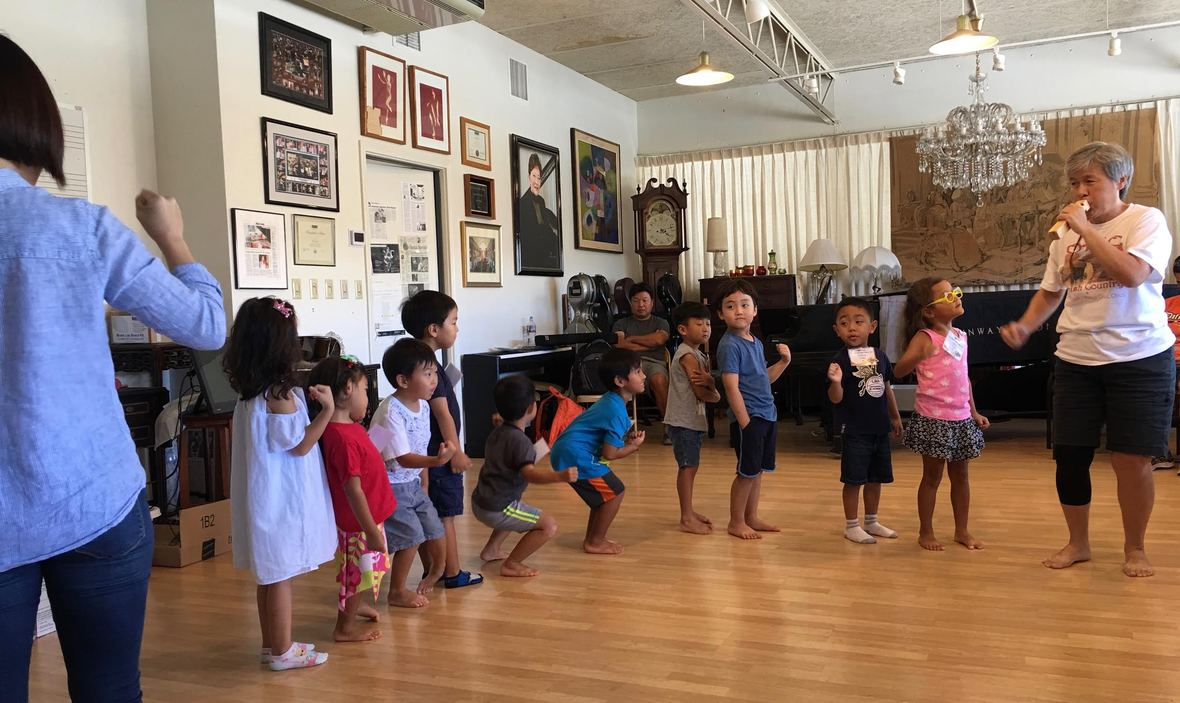/* styles */ Youngsters in Dr. Chet-Yeng Loong’s Early Childhood Music Program enjoy listening to music and following musical instruction as Dr. Loong leads them through various activities during class. If you would like your child to benefit from her extensive experience working with young children, indirectly training them to understand musical concepts, a new class for children ages 4-5 will begin in January 2018. Classes will be held on Saturdays from 1:20-2:20pm. Class size is limited to 10 children. Cost is \$165 for 10 sessions. Call Masaki School of Music at 593-9395 or email masakischoolofmusic@gmail.com if you are interested in signing your child up for this class.
 table div table+table+table+table+table+table+table+table+table+table+table+table+table+table+table+table+table+table+table+table+table+table+table+table div table{width:100%;padding:0}table div table+table+table+table+table+table+table+table+table+table+table+table+table+table+table+table+table+table+table+table+table+table+table+table div table img{width:96.23%;padding:0;float:none}table div table+table+table+table+table+table+table+table+table+table+table+table+table+table+table+table+table+table+table+table+table+table+table+table div table td{width:100%;padding:0 1.88% 18px}/* styles */## 35th Ala Moana CenterStage Christmas Extravaganza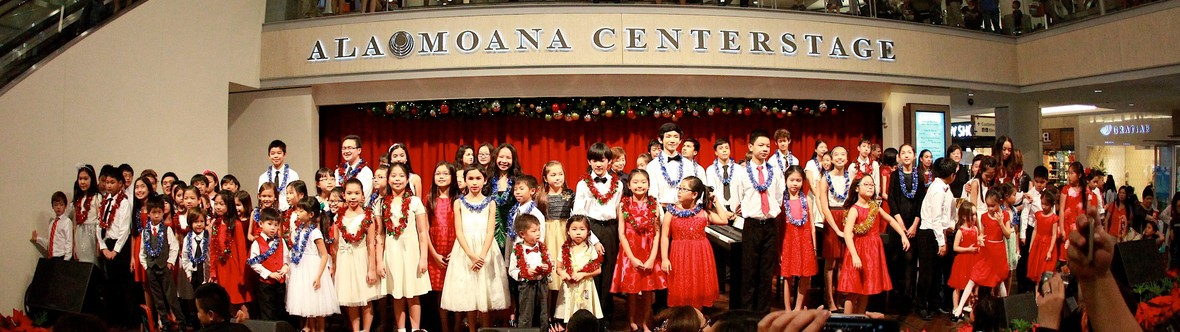/* styles */ On Sunday, December 17, 2017 at 7:00pm students from the Masaki School of Music will participate in our 35th Ala Moana Centerstage Christmas Extravaganza. Rehearsals will be starting soon and the recital room will be filled with 12 digital pianos in preparation for our students to begin piano ensemble rehearsals. A performance not to be missed, be sure to arrive early to find a spot to view this holiday highlight. (Photo is from our 2016 performance.)
 table div table+table+table+table+table+table+table+table+table+table+table+table+table+table+table+table+table+table+table+table+table+table+table+table+table+table+table+table div table{width:100%;padding:0}table div table+table+table+table+table+table+table+table+table+table+table+table+table+table+table+table+table+table+table+table+table+table+table+table+table+table+table+table div table img{width:96.23%;padding:0;float:none}table div table+table+table+table+table+table+table+table+table+table+table+table+table+table+table+table+table+table+table+table+table+table+table+table+table+table+table+table div table td{width:100%;padding:0 1.88% 18px}/* styles */## Holiday Concert Series at Kahala Mall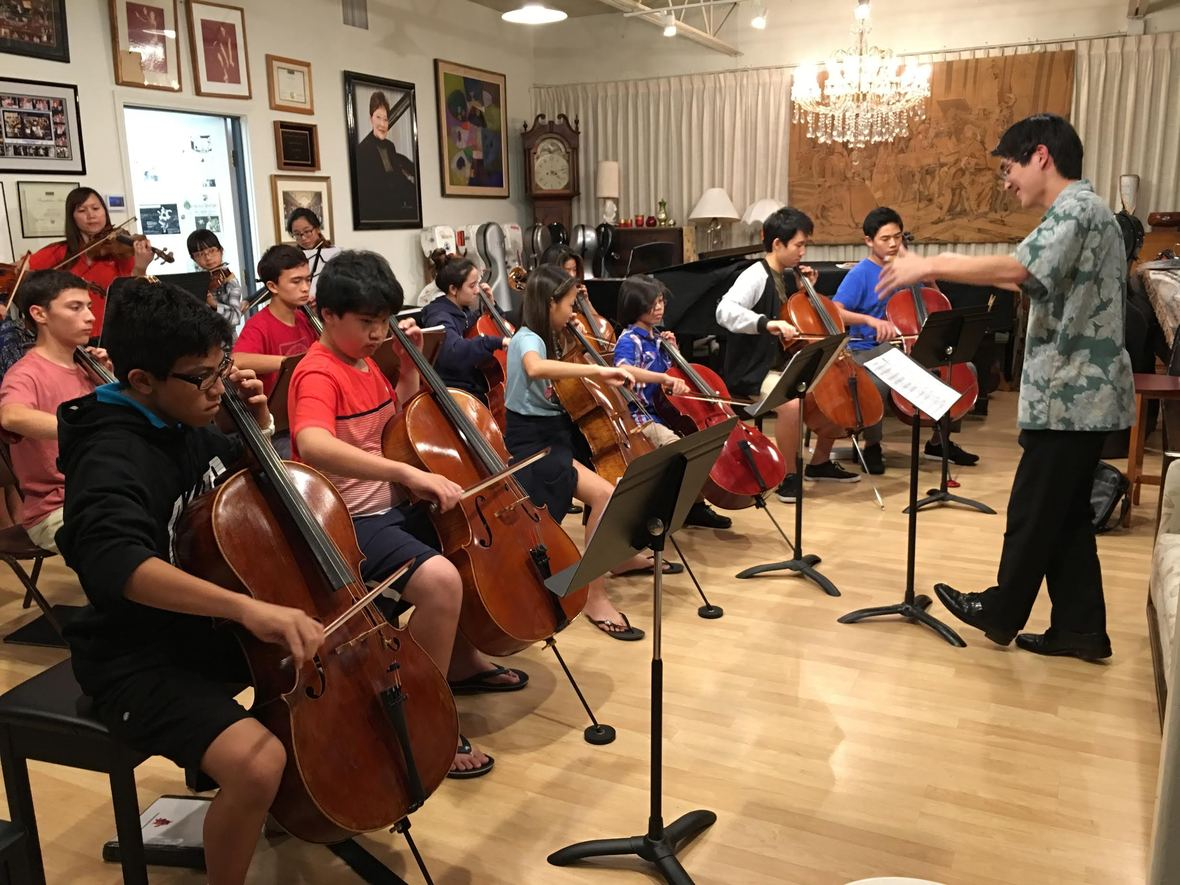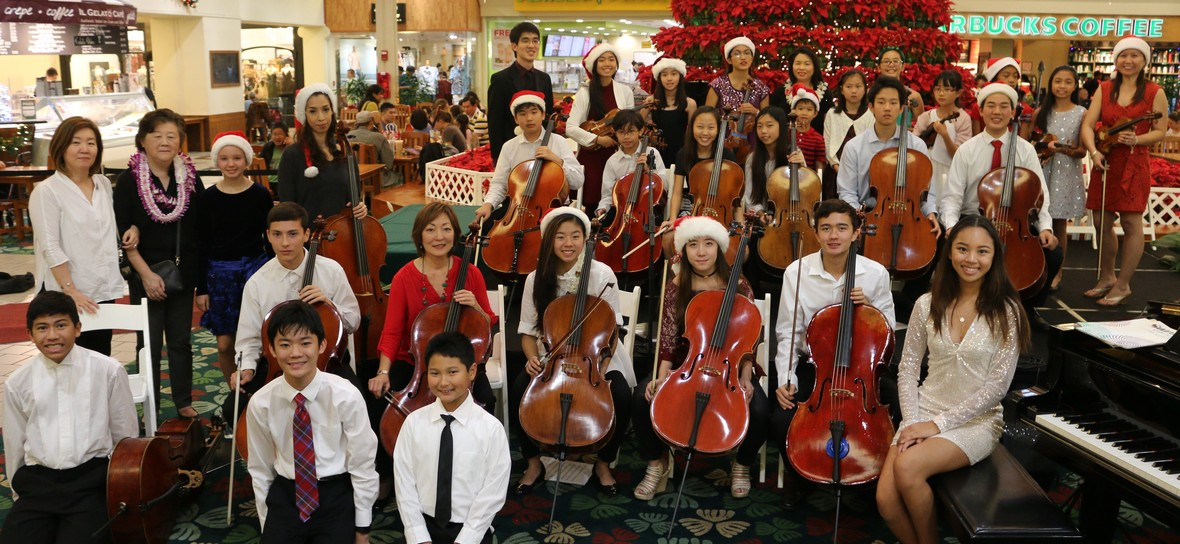/* styles */ On Friday, December 15, 2017, at 5:00pm students from the Masaki School of Music will perform at the Kahala Mall. String ensembles and piano soloists will perform Holiday as well as Classical favorites. Shoppers stop in their tracks to enjoy the musical performances by our talented young musicians. (Photos of string ensemble rehearsal and at Kahala Mall in December 2016.)
 table div table+table+table+table+table+table+table+table+table+table+table+table+table+table+table+table+table+table+table+table+table+table+table+table+table+table+table+table+table+table+table+table div table{width:100%;padding:0}table div table+table+table+table+table+table+table+table+table+table+table+table+table+table+table+table+table+table+table+table+table+table+table+table+table+table+table+table+table+table+table+table div table img{width:96.23%;padding:0;float:none}table div table+table+table+table+table+table+table+table+table+table+table+table+table+table+table+table+table+table+table+table+table+table+table+table+table+table+table+table+table+table+table+table div table td{width:100%;padding:0 1.88% 18px}/* styles */## Masaki School of Music welcomes our new instructors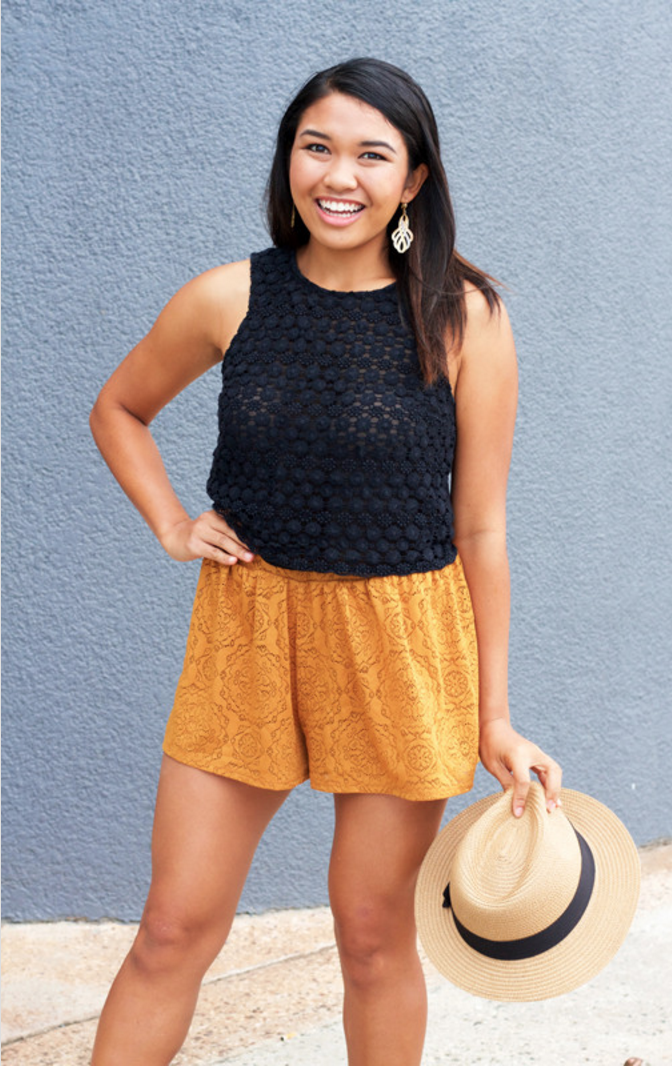Teaching voice is a recent U.H. graduate, Brooke Alcuran. A student of Dr. Maya Hoover, Brooke is skilled at working with children, as young as 4 years old. If your child would like to sing in a small group chorus, Brooke has the skills and the background to make this an exciting learning experience. Private lessons are also available for students of any age.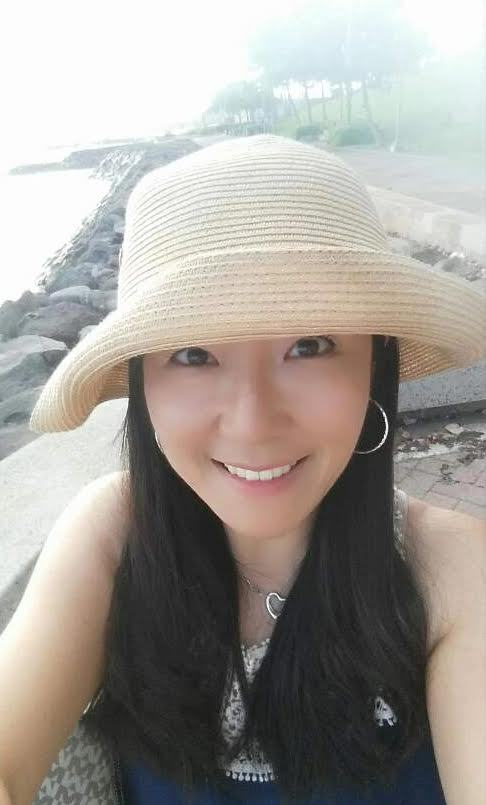Teaching voice and piano is Sharon Soo-Yeun Myung. Sharon is currently a lecturer at Leeward Community College where she teaches voice and coaches the college chorus. Her vocal and piano training were predominantly completed in Korea and at the Ewha Womans’ University.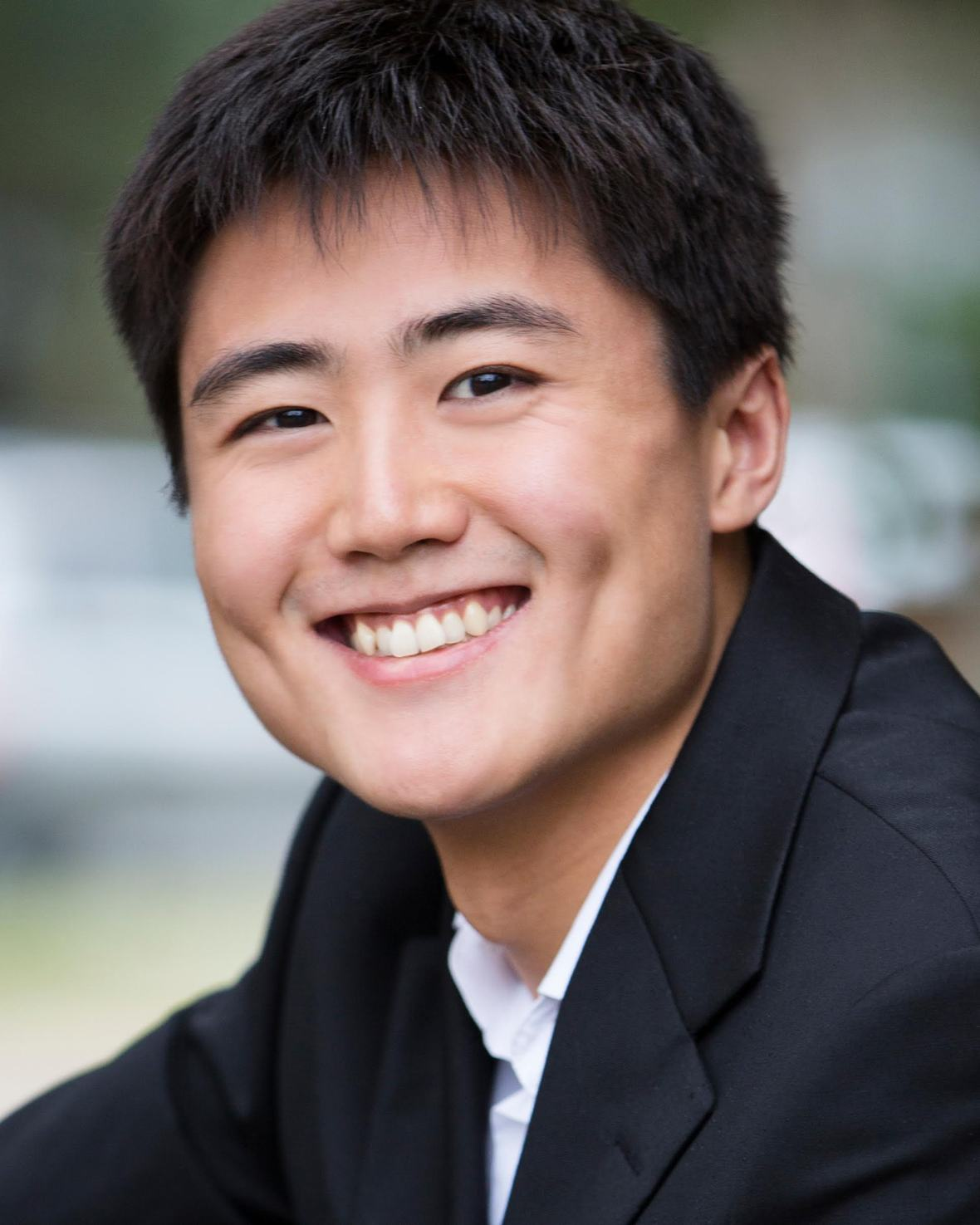Returning to Honolulu to pursue his Master’s in Music Performance at the UH-Manoa is former student of Ellen Masaki, Irwin Jiang. Irwin was a student of Mrs. Masaki for 11 years prior to attending the College of Charleston in South Carolina on a full scholarship. He was a recipient of the Morning Music Club Scholarship and was a performer on National Public Radio’s "From the Top" while studying with Ellen Masaki.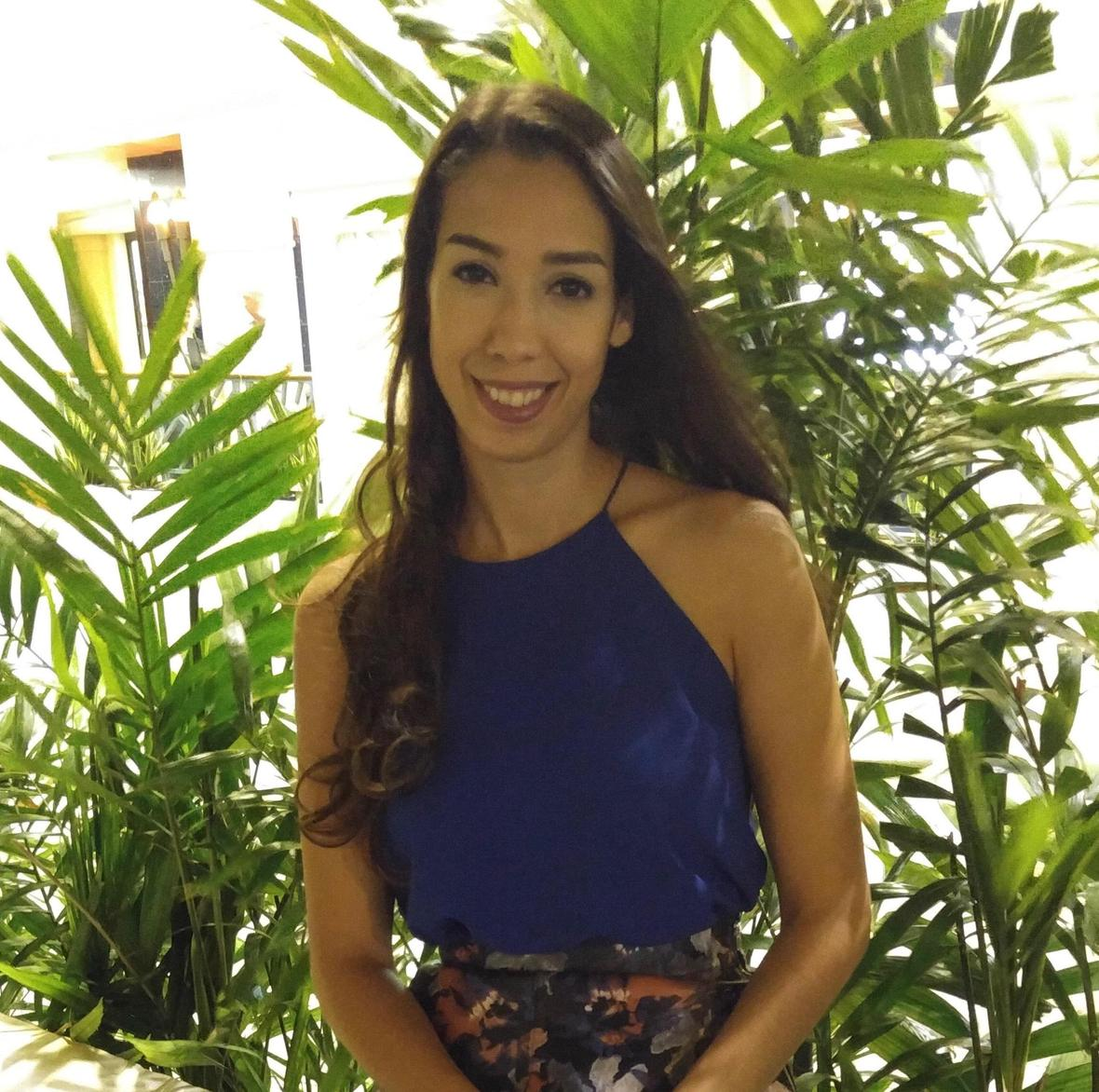Cellist, Tugce Bryant, joined the Masaki School of Music in the summer of 2016. She received her master’s degree in violoncello performance from Valdosta State University and played with various symphony orchestras in Georgia and Florida. Besides teaching cello at the MSM, Tugce plays with the Hawaii Symphony Orchestra.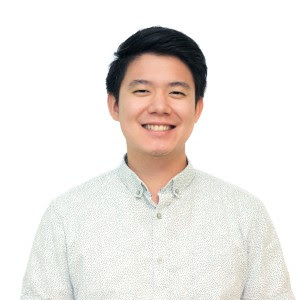Cellist, Aris Doike joined Masaki School of Music in March of 2017. Aris was born and raised in Honolulu and is a graduate of Moanalua High School. He received his Bachelor of Music Degree from the University of Colorado at Boulder and a Performer’s Diploma from Southern Methodist University. A recipient of the Jack Kent Cooke Scholarship from National Public Radio’s “From the Top” and the Theodore Presser Scholarship, Aris was recently appointed, Operations Manager for the Hawaii Youth Symphony. He also performs with the Hawaii Symphony Orchestra.

 table div table+table+table+table+table+table+table+table+table+table+table+table+table+table+table+table+table+table+table+table+table+table+table+table+table+table+table+table+table+table+table+table+table+table+table+table+table+table+table div table{width:100%;padding:0}table div table+table+table+table+table+table+table+table+table+table+table+table+table+table+table+table+table+table+table+table+table+table+table+table+table+table+table+table+table+table+table+table+table+table+table+table+table+table+table div table img{width:96.23%;padding:0;float:none}table div table+table+table+table+table+table+table+table+table+table+table+table+table+table+table+table+table+table+table+table+table+table+table+table+table+table+table+table+table+table+table+table+table+table+table+table+table+table+table div table td{width:100%;padding:0 1.88% 18px}/* styles */## Musical Accomplishments

 table div table+table+table+table+table+table+table+table+table+table+table+table+table+table+table+table+table+table+table+table+table+table+table+table+table+table+table+table+table+table+table+table+table+table+table+table+table+table+table+table+table div table td,table.module-40{width:100%;padding:0}table div table+table+table+table+table+table+table+table+table+table+table+table+table+table+table+table+table+table+table+table+table+table+table+table+table+table+table+table+table+table+table+table+table+table+table+table+table+table+table+table+table div table{width:100%;float:none;margin-left:auto;margin-right:auto;padding:0}table div table+table+table+table+table+table+table+table+table+table+table+table+table+table+table+table+table+table+table+table+table+table+table+table+table+table+table+table+table+table+table+table+table+table+table+table+table+table+table+table+table div table a{border:0 none;text-decoration:none}table div table+table+table+table+table+table+table+table+table+table+table+table+table+table+table+table+table+table+table+table+table+table+table+table+table+table+table+table+table+table+table+table+table+table+table+table+table+table+table+table+table div table img{width:100%!important;border:0 none;text-decoration:none}/* styles */
 /* styles */ Noe Baladad, instructor at MSM and a student of Dr. Tommy Yee at the U.H., performed in Carnegie Hall in NY on October 14th. The competition Noe entered is titled the American Fine Arts Festival (Golden Era of Romantic Music International Competition 2017). Noe was 1 of 4 awarded first place (the other three were from South Korea, Japan, and Germany). As a result, Noe was invited to perform (for the second time) at Carnegie Hall’s Weill Recital Hall and has been invited to study abroad in the AFAF Summer Festival in Europe in 2018. She will be traveling to Germany, Austria, and Italy. One of the highlights of the tour will be performing at the Robert Schumann Museum in Zwickau, Germany. Congratulations to Noe!
 table div table+table+table+table+table+table+table+table+table+table+table+table+table+table+table+table+table+table+table+table+table+table+table+table+table+table+table+table+table+table+table+table+table+table+table+table+table+table+table+table+table+table+table div table{width:100%;padding:0}table div table+table+table+table+table+table+table+table+table+table+table+table+table+table+table+table+table+table+table+table+table+table+table+table+table+table+table+table+table+table+table+table+table+table+table+table+table+table+table+table+table+table+table div table img{width:96.23%;padding:0;float:none}table div table+table+table+table+table+table+table+table+table+table+table+table+table+table+table+table+table+table+table+table+table+table+table+table+table+table+table+table+table+table+table+table+table+table+table+table+table+table+table+table+table+table+table div table td{width:100%;padding:0 1.88% 18px}/* styles */## Honolulu Chamber Music presents Stephen Hough in recital tonight!

 table.module-45{width:92.08%;padding:0}table div table+table+table+table+table+table+table+table+table+table+table+table+table+table+table+table+table+table+table+table+table+table+table+table+table+table+table+table+table+table+table+table+table+table+table+table+table+table+table+table+table+table+table+table+table+table div table{width:92.08%;float:none;margin-left:auto;margin-right:auto;padding:0}table div table+table+table+table+table+table+table+table+table+table+table+table+table+table+table+table+table+table+table+table+table+table+table+table+table+table+table+table+table+table+table+table+table+table+table+table+table+table+table+table+table+table+table+table+table+table div table a{border:0 none;text-decoration:none}table div table+table+table+table+table+table+table+table+table+table+table+table+table+table+table+table+table+table+table+table+table+table+table+table+table+table+table+table+table+table+table+table+table+table+table+table+table+table+table+table+table+table+table+table+table+table div table img{width:100%!important;border:0 none;text-decoration:none}table div table+table+table+table+table+table+table+table+table+table+table+table+table+table+table+table+table+table+table+table+table+table+table+table+table+table+table+table+table+table+table+table+table+table+table+table+table+table+table+table+table+table+table+table+table+table div table td{width:100%;padding:0}/* styles */
 /* styles */ This is one of THE piano events to see in Honolulu this year!! Here are a couple fun recordings if you're not already familiar with Stephen Hough's playing (his own arrangement of My Favorite Things and MacDowell's Hexantanz). Purchase tickets through The University of Hawai‘i at Mānoa, Music Department here
 table div table+table+table+table+table+table+table+table+table+table+table+table+table+table+table+table+table+table+table+table+table+table+table+table+table+table+table+table+table+table+table+table+table+table+table+table+table+table+table+table+table+table+table+table+table+table+table+table div table{width:100%;padding:0}table div table+table+table+table+table+table+table+table+table+table+table+table+table+table+table+table+table+table+table+table+table+table+table+table+table+table+table+table+table+table+table+table+table+table+table+table+table+table+table+table+table+table+table+table+table+table+table+table div table img{width:96.23%;padding:0;float:none}table div table+table+table+table+table+table+table+table+table+table+table+table+table+table+table+table+table+table+table+table+table+table+table+table+table+table+table+table+table+table+table+table+table+table+table+table+table+table+table+table+table+table+table+table+table+table+table+table div table td{width:100%;padding:0 1.88% 18px}/* styles */## Hawaii Symphony Orchestra and Hawaii Youth Symphony’s Side-By-Side concert

 table div table+table+table+table+table+table+table+table+table+table+table+table+table+table+table+table+table+table+table+table+table+table+table+table+table+table+table+table+table+table+table+table+table+table+table+table+table+table+table+table+table+table+table+table+table+table+table+table+table+table div table td,table.module-49{width:100%;padding:0}table div table+table+table+table+table+table+table+table+table+table+table+table+table+table+table+table+table+table+table+table+table+table+table+table+table+table+table+table+table+table+table+table+table+table+table+table+table+table+table+table+table+table+table+table+table+table+table+table+table+table div table{width:100%;float:none;margin-left:auto;margin-right:auto;padding:0}table div table+table+table+table+table+table+table+table+table+table+table+table+table+table+table+table+table+table+table+table+table+table+table+table+table+table+table+table+table+table+table+table+table+table+table+table+table+table+table+table+table+table+table+table+table+table+table+table+table+table div table a{border:0 none;text-decoration:none}table div table+table+table+table+table+table+table+table+table+table+table+table+table+table+table+table+table+table+table+table+table+table+table+table+table+table+table+table+table+table+table+table+table+table+table+table+table+table+table+table+table+table+table+table+table+table+table+table+table+table div table img{width:100%!important;border:0 none;text-decoration:none}/* styles */
 /* styles */ Hawaii Youth Symphony’s top orchestra, Youth Symphony I (YSI), will perform with the Hawai‘i Symphony Orchestra (HSO) at the Blaisdell Concert Hall on Thursday November 2 at 7:30 pm. Each HSO musician will sit next to a YSI musician, making for an unforgettable evening for the youth symphony musicians, Hawai‘i Symphony musicians and the audience. Many HSO musicians are private teachers to HYS students or are youth symphony alumni themselves—this will be a very special opportunity! The 2017-2018 edition of YSI is composed of 103 of Hawaii’s top high school students, grades 9-12 and conducted by Joseph Stepec. The Hawaii Symphony Orchestra musicians will perform Dmitri Shostakovich’s Festive Overture, Op. 96. The side-by-side group will perform John Corigliano’s Mannheim Rocket, John Williams’ Yoda’s Theme from Star Wars and Jean Sibelius’ Finale from Symphony No. 2 in D Major, Op. 43. Tickets: Tickets are general admission, \$20 for adults and \$10 for students, through the Hawai‘i Symphony Orchestra Box Office available online here, or by calling 94-MUSIC (946-8742).
 table div table+table+table+table+table+table+table+table+table+table+table+table+table+table+table+table+table+table+table+table+table+table+table+table+table+table+table+table+table+table+table+table+table+table+table+table+table+table+table+table+table+table+table+table+table+table+table+table+table+table+table+table div table{width:100%;padding:0}table div table+table+table+table+table+table+table+table+table+table+table+table+table+table+table+table+table+table+table+table+table+table+table+table+table+table+table+table+table+table+table+table+table+table+table+table+table+table+table+table+table+table+table+table+table+table+table+table+table+table+table+table div table img{width:96.23%;padding:0;float:none}table div table+table+table+table+table+table+table+table+table+table+table+table+table+table+table+table+table+table+table+table+table+table+table+table+table+table+table+table+table+table+table+table+table+table+table+table+table+table+table+table+table+table+table+table+table+table+table+table+table+table+table+table div table td{width:100%;padding:0 1.88% 18px}/* styles *//* styles */ web: MasakiSchoolofMusic.com FB MasakiSchoolOfMusic E: masakischoolofmusic@gmail.com Address: 250 Ward Avenue, Suite 226, Honolulu, HI 96814 Phone: (808) 593-9395